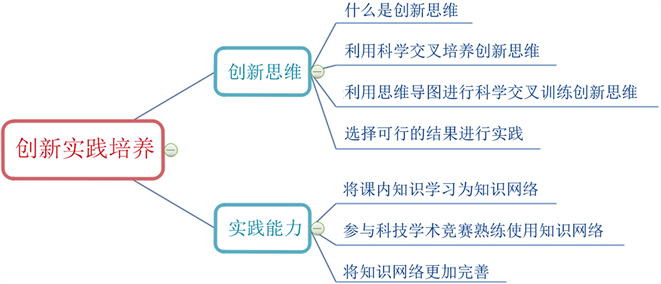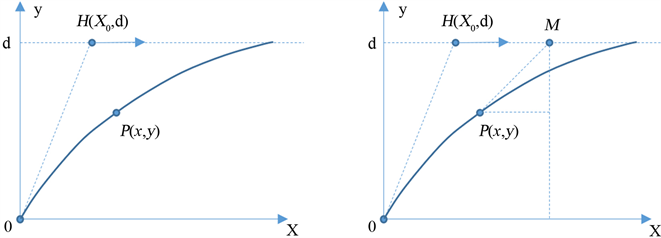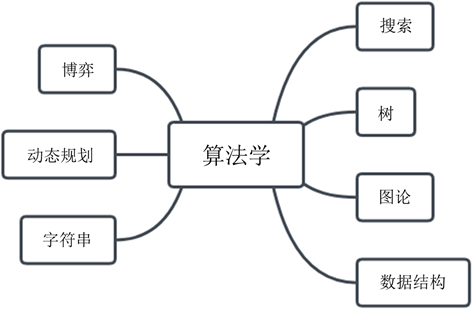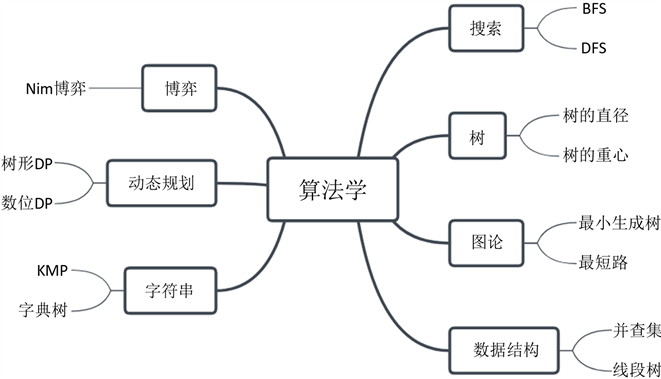# 基于思维导图的实践创新能力培养方法研究与实践Research and Practice on Cultivation Method of Practical Innovation Ability Based on Thought Guide Map

• 全文下载: PDF(1567KB)    PP.166-173   DOI: 10.12677/CES.2019.72030
• 下载量: 286  浏览量: 515   科研立项经费支持

Students’ ability to practice innovation is an extremely important aspect in the training of science and engineering talents. Starting from the innovative thinking, this paper proposes to break through the inherent flaws of each discipline through interdisciplinary and use the mind map to make reasonable innovation from a scientific point of view. In the following practice, the knowledge network using the root-seeking method will be easier to grasp and use, and the mind map can be further improved to form a virtuous circle. Next, the effectiveness and feasibility of the method are verified by analyzing the examples of innovation practice in the ACM algorithm competition.

1. 引言

2. 创新实践培养方法

2.1. 创新思维方面

2.2. 如何训练创新思维2.3. 在实践能力方面

2.4. 如何提高实践能力(a) (b)

Figure 2. Missile trajectory and aircraft carrier position

${\left(\frac{\text{d}x}{\text{d}t}\right)}^{2}+{\left(\frac{\text{d}y}{\text{d}t}\right)}^{2}={v}_{D}^{2}$ (1)

${v}_{H}=32节=32×1.852\text{\hspace{0.17em}}\text{km}/\text{h}=\frac{7408}{125}\text{\hspace{0.17em}}\text{km}/\text{h}\approx 59.264\text{\hspace{0.17em}}\text{km}/\text{h}$

$\frac{\text{d}y}{\text{d}x}=\frac{d-y}{{v}_{H}t-x}$ (2)

$\frac{\text{d}y}{\text{d}x}=\frac{\text{d}x}{\text{d}t}\left(\frac{d-y}{{v}_{H}t-x}\right)$ (3)

$\left\{\begin{array}{l}{\left(\frac{\text{d}x}{\text{d}t}\right)}^{2}+{\left(\frac{\text{d}y}{\text{d}t}\right)}^{2}={v}_{D}^{2}\\ \frac{\text{d}y}{\text{d}x}=\frac{\text{d}x}{\text{d}t}\left(\frac{d-y}{{v}_{H}t-x}\right)\end{array}$ (4)

$\frac{\text{d}x}{\text{d}y}\left(d-y\right)={v}_{H}t-x$

$\frac{{\text{d}}^{2}x}{\text{d}{y}^{2}}\frac{\text{d}y}{\text{d}t}\left(d-y\right)+\frac{\text{d}x}{\text{d}y}\left(-\frac{\text{d}y}{\text{d}t}\right)={v}_{H}-\frac{\text{d}x}{\text{d}t}$

$\frac{{\text{d}}^{2}x}{\text{d}{y}^{2}}\frac{\text{d}y}{\text{d}x}\left(d-y\right)={v}_{H}$

${\left(\frac{\text{d}y}{\text{d}t}\right)}^{2}+\left({\left(\frac{\text{d}x}{\text{d}y}\right)}^{2}+1\right)={v}_{D}^{2}⇒\frac{\text{d}y}{\text{d}t}=\frac{{v}_{D}}{\sqrt{{\left(\frac{\text{d}x}{\text{d}y}\right)}^{2}+1}}$

$\left\{\begin{array}{l}\frac{{\text{d}}^{2}x}{\text{d}{y}^{2}}\cdot \frac{\left(d-y\right)}{\sqrt{{\left(\frac{\text{d}x}{\text{d}y}\right)}^{2}+1}}=\frac{{v}_{H}}{{v}_{D}}\text{ }\text{ }\text{ }\left(5\right)\\ {\frac{\text{d}x}{\text{d}y}|}_{y=0}=0\left(6\right)\\ {x|}_{y=0}=0\text{ }\left(7\right)\end{array}$

2.5. 在ACM算法程序设计竞赛中进行创新实践的实例

${a}^{b}$Figure 3. Simple mind map of algorithmologyFigure 4. Mind map of algorithmology

$11=b=1×{2}^{0}+1×{2}^{1}+0×{2}^{2}+1×{2}^{3}$

${a}^{11}={a}^{{2}^{0}}+{a}^{{2}^{1}}+{a}^{{2}^{3}}$

3. 总结

  钟经文. 创新, 第一动力引领全面发展[N]. 经济日报, 2018-12-14(001).  郑婧. 大学生自主创新能力培养研究[D]: [硕士学位论文]. 西安: 西北工业大学, 2007.  吴静. 基于思维导图培养学生创新思维的教学模式研究[D]: [硕士学位论文]. 石家庄: 河北师范大学, 2012.  叶晓雁, 谢蕾, 杨小玲. 加强科学方法论教育提高实践与创新能力[J]. 北京邮电大学学报(社会科学版), 2000(2): 49-51.  周守仁. 前沿科学方法论与创新思维[J]. 江苏科技大学学报(社会科学版), 2004(4): 1-7.  李世利. 打破“思维定式”转变思路才有出路[N]. 沈阳日报, 2018-11-20(001).  苏勇林, 张建兵, 戴睿, 郭荣辉, 王孝军, 陈慧. 跨学科创新人才培养模式探索与实践[J]. 皮革科学与工程, 2019, 29(1): 76-80.  王敏, 王银玲, 阎世梁, 熊亮. 基于学科交叉的复合创新人才培养研究[J]. 高校实验室工作研究, 2018(4): 118-120.  龚玉. 基于学科交叉的高校国家重点实验室研究[D]: [硕士学位论文]. 上海: 华东师范大学, 2010.  李传秀, 张学辉, 钟敏. 思维导图在程序设计教与学中的应用探究[J]. 教育教学论坛, 2019(5): 165-166.  张艳霞. 使用思维导图支持探究性学习教学案例研究[D]: [硕士学位论文]. 北京: 首都师范大学, 2006.  叶婉婷. 思维导图在大学生协作学习中的行动研究[D]: [硕士学位论文]. 宁波: 宁波大学, 2007.  商宪丽. 基于主题引用网络的交叉学科知识传播研究——以数字图书馆为例[J]. 情报科学, 2018, 36(8): 53-59.  常芷玉, 王虹玲. 浅谈大学生社会创新实践能力培养的重要性[J]. 才智, 2019(3): 35.  赛氪: 2018年第八届MathorCup高校数学建模挑战赛通知[R]. https://www.saikr.com/vse/mathorcup/2018, 2019-02-24  Wikipedia (2019) International Collegiate Programming Contest. https://en.wikipedia.org/wiki/International_Collegiate_Programming_Contest  Wikipedia (2019) B+ tree. https://en.wikipedia.org/wiki/B%2B_tree  柯善杰, 魏志超, 陈玉前, 等. 大学生创新训练项目培养学生创新能力的研究实践[J]. 教育教学论坛, 2015(44): 120-121.